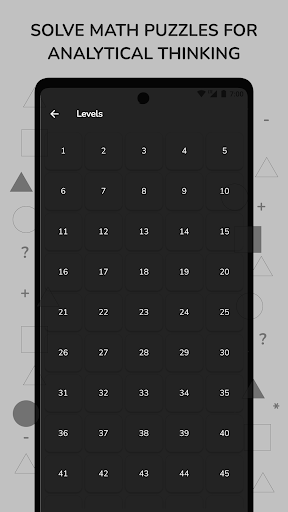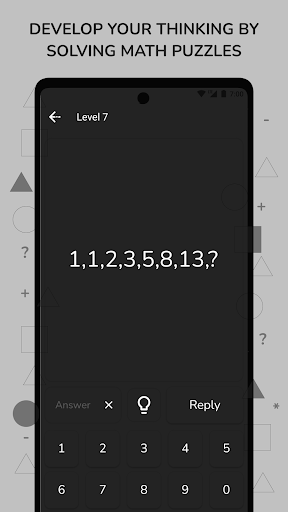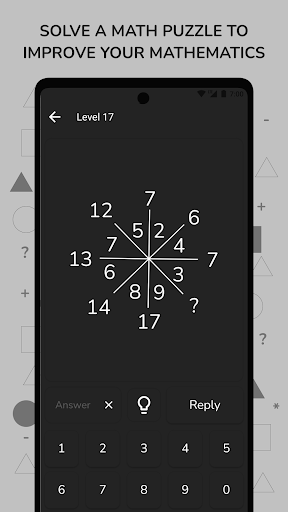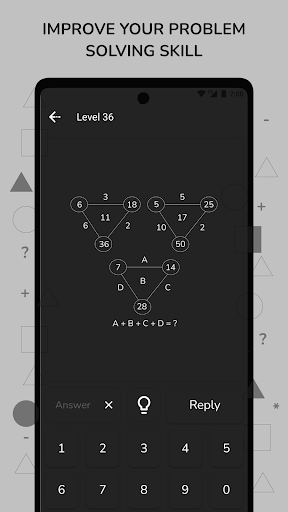# Math Puzzles & Brain Riddles

0.0

##### About Math Puzzles & Brain Riddles
Solving Math puzzles and Math Riddles allows you to make fast and accurate decisions. Solving a Mathematical Puzzle improves analytical thinking and allows us to use both sides of our brain. Math Puzzles and Riddles improve people of all ages. All questions of this math study game contain math tricks with answers, hints, and Math Solutions to solve Math Riddles. Math solutions make mental math problems very easy. Playing tricky Puzzle games will improve knowledge, memory, and logic.

Solving Math puzzles and riddles can be a fun and engaging way to learn and practice mathematical concepts. By engaging both sides of the brain, these types of games can help improve analytical thinking, decision-making skills, and logical reasoning ability. In addition to the cognitive benefits, solving Math puzzles and riddles can also provide a sense of accomplishment and boost self-confidence.

Math puzzles and riddles come in many different forms and can focus on a variety of mathematical concepts. Some examples include logic puzzles that require logical reasoning to solve, mental math challenges that test calculation skills, and word problems that apply mathematical concepts to real-world scenarios. Riddles that use mathematical language and concepts to create a puzzle or joke are another popular type of Math puzzle.

Educational games are very knowledge-enhancing for students. Mathematics is of course fun for easy learners. Here Math Puzzles are the first step to learning Math easily.

Benefits of solving Maths Puzzles
📍It will increase your processing speed.
📍Brain teasers strengthen memory.
📍Math Puzzles give you different thinking.
📍Math Riddle games help increase logical reasoning ability.
📍Logic games improve mental math calculation.
📍Logical reasoning math games, Solving Math puzzles increase the IQ level.
📍It increases the speed of learning and comprehension.

To talk more about the benefits of Math Puzzles. Since solving Maths Puzzles forces our brains, the development will be very fast.
Math Riddles enable quick and solution-oriented thinking.
Each of the math questions has a different solution and focuses on finding them and improving yourself. Solving Math Puzzles keeps the mental age young. Math Riddles allows you to do quick calculations. You will improve your quick problem-solving skills. Solving Mathematics Puzzles gives people self-confidence. The main goal of Math Puzzles is to make people think faster and more logically.

In addition to the cognitive benefits, solving Math puzzles and riddles can also provide a sense of accomplishment and boost self-confidence. These types of games can be a great way to learn and practice math in a fun and engaging way.

Contents of Math Puzzle

Math puzzles are a fun and engaging way to improve your mathematical skills and critical thinking abilities. With 100 unique and challenging questions, this Math puzzle game offers a range of difficulties and special formulas to solve. As you progress through the game, your progress will be recorded and the mathematical operations will become more difficult.

To solve the puzzles, players can use their analytical skills and logical reasoning to find the formulas specific to each question. For added support, the game also offers hints to help players when they get stuck. The questions are thought-provoking and designed to challenge players of all levels.

In addition to the cognitive benefits, this Math puzzle game also offers a simple and user-friendly interface that is easy on the eyes. Players can enjoy hours of engaging and educational gameplay without straining their eyes or attention span.

Overall, this Math puzzle game is a great way to improve your mathematical skills, critical thinking abilities, and overall brain power. Whether you are a beginner or an advanced player, this game offers something for everyone. Give it a try and see how far you can go!##### Recent Updates - Version 2.0.0
• Question 45 and 48 Edited